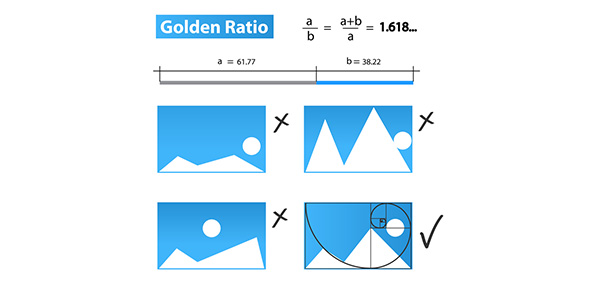# Ratios And Unit Rates

10 Questions | Attempts: 1948
ShareSettings• 1.
A comparison of two quantities or numbers
• A.

Proportion

• B.

Ratio

• C.

Unit

• D.

Unit rate

• 2.
comparing two different variables:  miles to gallons, sandwiches to people, etc.
• A.

Proportion

• B.

Rate

• C.

Ratio

• D.

Unit

• 3.
Finding how much ONE quantity would be           (per gallon, per ounce, per person)
• A.

Proportion

• B.

Ratio

• C.

Unit

• D.

Unit rate

• 4.
Two ratios that are equivalent.
• A.

Proportion

• B.

Ratio

• C.

Unit rate

• D.

Rate

• 5.
Equivalent
• A.

Various

• B.

Total

• C.

Sum

• D.

Equal

• 6.
Putting a fraction or ratio in LOWEST TERMS
• A.

Simplify

• B.

Reduce

• C.

Make small

• D.

None of the above

• 7.
Which is NOT the correct way to say a ratio?
• A.

1:4

• B.

1 to 4

• C.

1 out of 4

• D.

One fourth

• 8.
Which ratio is equivalent to 3:4?
• A.

9:12

• B.

6:12

• C.

12:9

• D.

None of the above

• 9.
What is the ratio 24:105 in lowest terms?
• A.

2:23

• B.

24:105

• C.

8:35

• D.

12:53

• 10.
Review:  Percent means out of...
• A.

1

• B.

10

• C.

100

• D.

1,000

## Related TopicsBack to top
×

Wait!
Here's an interesting quiz for you.